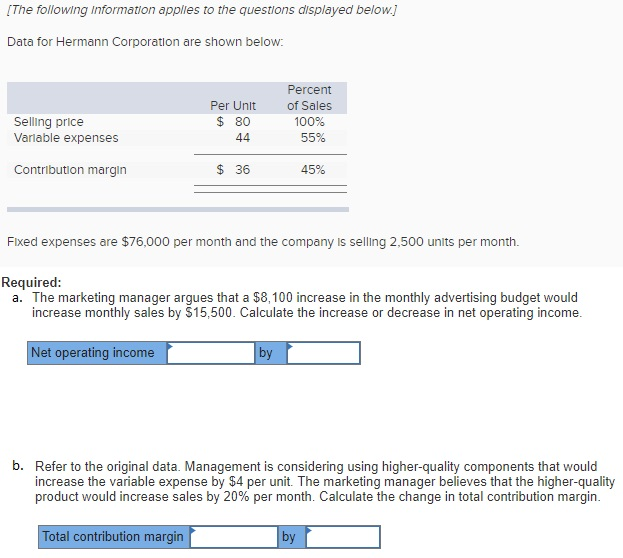# Question & Answer: Data for Hermann Corporation are shown below: Fixed expenses are \$76,000 per month and the company is…..Data for Hermann Corporation are shown below: Fixed expenses are \$76,000 per month and the company is selling 2.500 units per month. Required: a. The marketing manager argues that a \$8, 100 increase in the monthly advertising budget would increase monthly sales by \$15, 500. Calculate the increase or decrease in net operating income. b. Refer to the original data. Management is considering using higher-quality components that would increase the variable expense by \$4 per unit. The marketing manager believes that the higher-quality product would increase sales by 20% per month. Calculate the change in total contribution margin.

Don't use plagiarized sources. Get Your Custom Essay on
Question & Answer: Data for Hermann Corporation are shown below: Fixed expenses are \$76,000 per month and the company is…..
GET AN ESSAY WRITTEN FOR YOU FROM AS LOW AS \$13/PAGE

a.Increase in contribution=(\$15500*45%)=\$6975

Less:increase in Fixed expenses=\$8100

Hence net operating income would decrease by=\$1125.

b.

Current contribution margin=(36*2500)=\$90000

Contribution margin/iunit would be=(\$80-(\$44+4))=\$32.

Hence total contribution margin would be=(32*(2500*120%)=\$96000.

Hence Total contribution margin would increase by=(\$96000-\$90000)=\$6000.